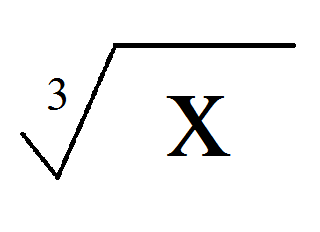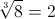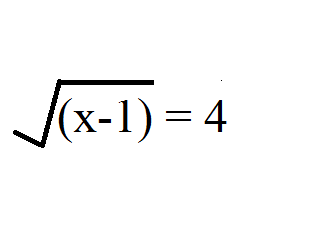# Solving Radical Equations: Steps and Examples

An error occurred trying to load this video.

Try refreshing the page, or contact customer support.

### You're on a roll. Keep up the good work!

Replay
Your next lesson will play in 10 seconds
• 0:27 What Is a Radical?
• 1:24 Inverse Operation
• 3:10 Extraneous Roots
• 4:40 What Does This Mean in…
• 6:28 Lesson Summary
Save Save

Want to watch this again later?

Timeline
Autoplay
Autoplay
Speed Speed

#### Recommended Lessons and Courses for You

Lesson Transcript
Instructor: Jennifer Beddoe

Jennifer has an MS in Chemistry and a BS in Biological Sciences.

Solving radical equations is not any more difficult than solving other algebraic equations. This lesson will show you how to solve equations containing a square root and give some real-world examples.

## It's Elementary, My Dear Watson

Sherlock Holmes is famous for his crime-solving abilities. No matter how small the clue, he could figure out 'who done it.' This was partly due to his detailed knowledge on a wide variety of topics.

Well, with some practice and knowledge of your own, you can become the Sherlock Holmes of algebra mysteries. You can solve for x no matter what the circumstances. This lesson will give you some of the knowledge that you need to become an algebra master.

What is a radical? You might be thinking (and rightly so) that a radical is someone who speaks out against injustices. They want to do things in a new and unconventional way. While this is true, it will not help at all with solving algebra problems.

For the purposes of this lesson, a radical is an algebraic term under a square root symbol. For example:It does not have to be a square root; it can be a cubed root, or fourth root or any other number. The square root is the most common and is what is implied when the radical symbol is used alone. If another root is called for, there will be a small superscript number in the 'v' of the root symbol, like this:The 3 indicates it is a cube root, which means that the term inside the radical equals a term that has been multiplied to itself three times. For example:This is correct because 2 * 2 * 2 = 8.

## Inverse Operations

Every mathematical operation has an inverse operation, an operation that is its opposite: for addition, it's subtraction; for multiplication, it's division; and for the square root, it's squared. Inverse operations are critical for solving algebraic equations.

For example, if you want to solve the following: x + 2 = 3. You need to get x alone. To do that, you perform the inverse operation, to move the 2 to the right side of the equation. That means, you subtract (the opposite of add) 2 from both sides of the equation. x + 2 = 3. Subtract 2 from both sides, and you get x = 1. And your problem is solved.

The method for solving equations containing radicals involves the same process. In order to isolate the x (or whatever the variable happens to be), you need to perform inverse operations to move all the numbers to the right side of the equation. It doesn't have to be the right side. That is just the most conventional way of doing things. You'll get the same answer either way.

Let's try an example. Solve for x:The first step to solving is to remove the radical symbol by performing the inverse operation, which is to square both sides. (√(x-1))^2 = 4^2. When you square the left side, the square and square root cancel each other out, so you are left with x - 1. On the right side, you simplify 4^2 = 16. Now, the problem is simple. x - 1 = 16. Just add one to both sides and the solution is x = 17.

## But Wait! There's More!

To unlock this lesson you must be a Study.com Member.

### Register to view this lesson

Are you a student or a teacher?

#### See for yourself why 30 million people use Study.com

##### Become a Study.com member and start learning now.
Back
What teachers are saying about Study.com

### Earning College Credit

Did you know… We have over 200 college courses that prepare you to earn credit by exam that is accepted by over 1,500 colleges and universities. You can test out of the first two years of college and save thousands off your degree. Anyone can earn credit-by-exam regardless of age or education level.